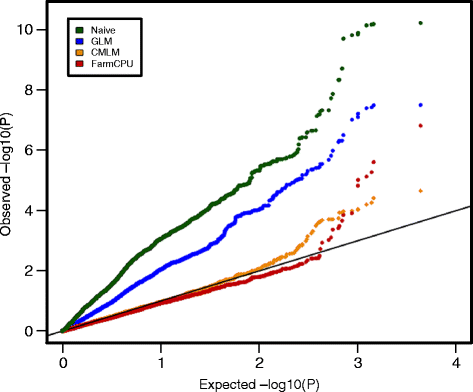# How to make Q-Q plots for different models at a single chart using R?

How to make Q-Q plots for different models at a single chart using R?

1

Hello everyone!
I am trying to make a Q-Q plot to visualise p-values obtained from different models afterr runing GWAS analysis. The key thing is that I want to reflect p-values from the different models in one chart. I tried qq function in qqman package however it takes only a vector of one model as an input, so as a result, I get the following picture:And what I am trying to should look like this:• 3.0k views

You could plot it outside any qq plotting functions. The following will generate the same graph:

Generate p-values:

``````p<- runif( 10000 )
``````

Using qqplot:

``````qqplot( -log10( ppoints(p ) ), -log10( p ) , col="red" )
``````

Using plot:

``````p.sorted <- sort(p)
plot( -log10(ppoints(p.sorted )), -log10(p.sorted) , col="red")
``````

(same as above). Then if you want to add another data set:

``````p2<- runif(5000)
p2.sorted <- sort(p2)
points( -log10(ppoints(p2.sorted )), -log10(p2.sorted) , col="blue" )
``````

You may have to play with the xlim and ylim argument of the plot function to include all values of subsequent datasets.